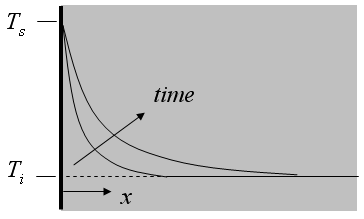Contents - Index

Constant Temperature StepThe function semiinf1(T_i,T_s,alpha,x,time) returns the temperature for a given position and time within a semi-infinite body exposed to a step change in the surface temperature using the relation provided in Table 3-2 of Nellis and Klein.

Inputs:
T_i - initial temperature of the solid  [C] or [K]
T_s - surface temperature subsequent to step [C] or [K]
alpha - thermal diffusivity [m^2/s]
x - perpendicular distance from surface [m]
time - time relative to step change (step occurred at t=0) [s]

This function can be used with English units set in EES.  In this case, T_i and DELTAT are in [F] or [R],  alpha is in ft^2/hr and internally converted to ft^2/s.  x is in [ft].  Time must be in s.

Example:
\$UnitSystem SI K Pa J
T_i=378[K]
T_s=303 [K]
alpha=0.00009547 [m^2/s]
x=0.05 [m]
time=2 [s]
T=semiinf1(T_i,T_s,alpha,x,time)

{Solution: T = T=377.2 [K]}

Transient Conduction Index